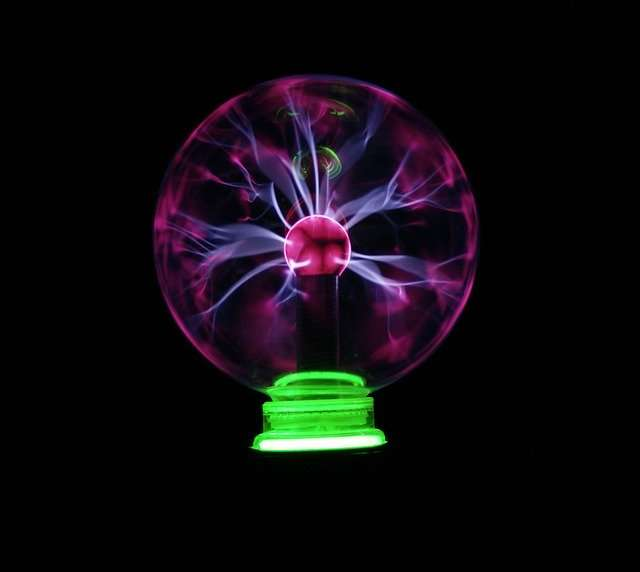# Impact of dielectric substances on alternative currentDielectric substances and insulators belong to the same material class with different origin and contexts. The two substances are primarily classified according to their use. The key difference is that dielectrics store the electrical charge in it and insulators are resistant to electron flow.

Comparison between the insulators and dielectrics

Note: Polarization property of the substance is the movement of negative and positive charges in the opposite directions.

## Dielectric used in capacitors to fulfil three functions

• To prevent the contact between the two conductive plates, a small palate (dielectrics) is placed between the two plates to separate them. The presence of dielectrics in the capacitors increases the capacitance
• Capacitance can be increased by reducing the strength of an electric field.
• To minimize the dielectric breakdown, during the high voltage operations.

The capacitance of the capacitor is calculated by using the formula.

Where

A = Area of the plates overlap each other

d = Distance between the plates

εr = Dielectric constant

## Electric Current

The electric current is defined as the flow rate of electrical charges (+ve and -ve charges) through the conducting medium.

When the conductive medium has a potential difference between the two regions, the electric charge starts to flow from the higher potential region to the lower potential region.

If the electric charge q coulomb is transferred between two regions with time t, then the current is

I = q/t

The unit of current is coulomb/sec.

## Types of current

(1)  Direct current – The current is called direct current when the current moves in one path either in a fixed or fluctuating way.

(2) Alternating current – When the current travels alternately in either path with frequency is known as alternating current.